﻿ Different Methods of Speed Control of Three-Phase Asynchronous Motor

### Different Methods of Speed Control of Three-Phase Asynchronous Motor

G. C. Diyoke, Okeke C., Uchechi Aniagwu

## Different Methods of Speed Control of Three-Phase Asynchronous Motor

G. C. Diyoke1,, Okeke C.1, Uchechi Aniagwu1

1Department of Electrical and Electronic Engineering, Michael Okpara University of Agriculture, Umudike Abia State, Nigeria

### Abstract

Asynchronous motors are the most widely used electrical motors due to their reliability, low cost, ruggedness and robustness. However, asynchronous motors do not inherently have the capability of variable speed operation. Due to this reason, earlier dc motors were applied in most of the electrical drives. But the recent developments in speed control methods of the asynchronous motor have led to their large scale use in almost all electrical drives. In this paper, various methods of speed control of induction (asynchronous) motors such as variable rotor resistance, variable stator voltage, constant Volts/hertz control, frequency variation, pole changing, etc., were carried out and MATLAB software was used to develop the codes for the analysis of these methods.

• G. C. Diyoke, Okeke C., Uchechi Aniagwu. Different Methods of Speed Control of Three-Phase Asynchronous Motor. American Journal of Electrical and Electronic Engineering. Vol. 4, No. 2, 2016, pp 62-68. http://pubs.sciepub.com/ajeee/4/2/3
• Diyoke, G. C., Okeke C., and Uchechi Aniagwu. "Different Methods of Speed Control of Three-Phase Asynchronous Motor." American Journal of Electrical and Electronic Engineering 4.2 (2016): 62-68.
• Diyoke, G. C. , C., O. , & Aniagwu, U. (2016). Different Methods of Speed Control of Three-Phase Asynchronous Motor. American Journal of Electrical and Electronic Engineering, 4(2), 62-68.
• Diyoke, G. C., Okeke C., and Uchechi Aniagwu. "Different Methods of Speed Control of Three-Phase Asynchronous Motor." American Journal of Electrical and Electronic Engineering 4, no. 2 (2016): 62-68.

 Import into BibTeX Import into EndNote Import into RefMan Import into RefWorks

123
Prev Next

### 1. Introduction

An induction or asynchronous motor is a type of AC motor where power is supplied to the rotor by means of electromagnetic induction, rather than a commutator or slip rings as in other types of motor. These motors are widely used in industrial drives, particularly poly-phase induction motors, because they are rugged and have no brushes. But they require much more complex methods of control, more expensive and higher rated power converters than DC and permanent magnet machines . Single-phase versions are used in small appliances. Their speed is determined by the frequency of the supply current, so they are most widely used in constant-speed applications, although variable speed versions, using variable frequency drives are becoming more common. The most common type is the squirrel cage motor, and this term is sometimes used for induction motors generally [2, 3, 4, 5]. But it is desirable to replace the single phase induction motor drives by three phase induction motor drives in residential appliances, farming and low power industrial applications. Induction motors have performed the main part of many speed control systems and found usage in several industrial applications . The benefit of improvement in the motor drive industry has touched varied applications, from heavy and large industrial equipment such as rolling mills in steel making plants, paper mills, etc. to “Mechatronics” equipment used in machine tools and semiconductor fabrication machines .

Three phase induction machines are synchronous speed machines, operating below synchronous speed when motoring and above synchronous speed when generating . They are comparatively less expensive to equivalent size synchronous or dc machines and range in size from a few watts to 10,000hp. As motors, they are rugged and require very little maintenance. However, their speeds are not as easily as with dc motors. They draw large starting currents, typically, about six to eight times their full load values, and operate with a poor lagging power factor when lightly loaded.

This paper is structured as: In section I, the concept of asynchronous motor and its applications. Section II presents different speed control techniques. Section III details the principle of operation of induction motor. Here in section IV presents in details the equivalent circuit analysis. In section V, Matlab/Simulink simulation results are displayed. Finally, in section VI conclusion is presented.

### 2. Different Speed Control Methods

The speed control of asynchronous motor (AM) or induction motor (IM) can be varied by varying the slip ‘S’ or number of poles ‘p’ or frequency ‘f’ of the supply. The ability of varying any one of the above three quantities will provide methods of speed control of an induction motor. Constant V/F method is commonly used for constant and variable speed control of induction motor. The different methods of speed control of IM can be broadly classified into scalar and vector control methods. In this paper, scalar control methods are used. The scalar methods of speed control can be classified as

A. Rotor Resistance Control

This method is applicable only to the wound rotor induction motors. Speed variation can be obtained by inserting external resistance in the rotor circuit. External resistances are placed in series with the rotor windings during starting to limit the starting current. Without the external resistances, the starting currents are many times the rated currents. Depending on the size of the machine, it can draw 300% to over 900% of full-load current. Once the motor is started, the external resistance can be cut out to obtain high torque throughout the accelerating range.

B. Supply Frequency Control

By changing the supply frequency, the motor synchronous speed can be altered and thus the torque-speed of a three- phase induction motor can be controlled. The synchronous speed of the motor at rated conditions is known as the base speed. By using variable frequency control, it is possible to adjust the speed of the motor either above or below the base speed. Increase in frequency increases the torque-speed relation and a decrease in frequency decreases the torque-speed relation of the motor.

C. Supply Voltage Control

As can be seen from Equation 12, the torque developed by an induction motor varies as square of the voltage applied to its stator terminals. Thus by varying the applied voltage, the electromagnetic torque developed by the motor can be varied. This method is generally used for small squirrel-cage motors where cost is an important criterion and efficiency is not. However, this method has rather limited range of speed control.

D. Stator Voltage and Frequency Control i.e. Volts per Hertz Control

The stator voltage and frequency control is to control the induction motor speed and torque by varying the ratio of voltage to frequency. When low voltage and low frequency is applied to the motor, the maximum torque available decreases at reduced speeds. If the ratio of voltage to frequency is kept constant, this technique allows the induction motor to deliver its rated torque at speeds up to its rated speed. This is because; the air gap flux is reduced due to the drop in the stator impedance while motor operates at a low frequency. We vary the stator voltage in such a way that the flux remains constant by simultaneously varying the supply frequency such that the ratio V/f remains constant .

E. Pole Changing

The primary factor in determining the speed of an induction motor is the number of poles, given by the formula;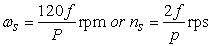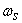= Synchronous speed, in revolutions per minute,

f = AC power frequency

p = Number of poles per phase winding.

From the formula above, Pole changing in induction machine can be done using a Dahlander motor also known as a pole changing motor. Pole changing can be used to achieve different speeds in induction machine by switching the configuration of the electrical stator windings in the ratio of 2:1, indirectly adding or removing poles and thus varying the rotor speed. The number of stator poles can be changed by (a) multiple stator winding, (b) method of consequent poles, (c) pole amplitude modulation.

### 3. Operating Principle

A three-phase power supply provides a rotating magnetic field in an induction motor. In both induction and synchronous motors, the AC power supplied to the motor's stator creates a magnetic field that rotates in time with the AC oscillations. Whereas a synchronous motor's rotor turns at the same rate as the stator field, an induction motor's rotor rotates at a slower speed than the stator field. The induction motor stator's magnetic field is therefore changing or rotating relative to the rotor. This induces an opposing current in the induction motor's rotor, in effect the motor's secondary winding, when the latter is short-circuited or closed through external impedance. The rotating magnetic flux induces currents in the windings of the rotor in a manner similar to currents induced in a transformer's secondary winding(s). The currents in the rotor windings in turn create magnetic fields in the rotor that react against the stator field. Due to Lenz's Law, the direction of the magnetic field created will be such as to oppose the change in current through the rotor windings. The cause of induced current in the rotor windings is the rotating stator magnetic field, so to oppose the change in rotor-winding currents; the rotor will start to rotate in the direction of the rotating stator magnetic field. The rotor accelerates until the magnitude of induced rotor current and torque balances the applied load. Since rotation at synchronous speed would result in no induced rotor current, an induction motor always operates slower than synchronous speed. Thus, the operation of induction motor results to losses and power output as show in Figure 1 below.Download asVeiw figureFigures index
Figure 1. Power flow diagram of 3-phase induction motor

The torque-slip/speed curve is drawn in Figure 2 below. This curve has the following characteristic features:Download asVeiw figureFigures index
Figure 2. Torque slip/speed curve of 3 phase induction motor

(1) When n=ns, and s=0, torque is zero. This is because at synchronous speed there is no relative motion because rotor conductors and stator rotating field, so that e. m. f and current are zero.

(2) The hatched area shows the normal operating range for motor action. In this region torque increases linearly with slip.

(3) CD represents the torque at s=1, i.e. at standstill conditions. If load torque is less than CD, the motor will accelerate till the torque developed by motor is equal to load torque. This happens at a speed which is close to but less than synchronous speed.

(4) AB is the maximum or pull-out torque. If the load torque is more than this torque, the motor will come to standstill. The region BD is the unstable region.

(5) The maximum torque occurs at a low value of slip. This is typical of all induction motors and is a desired feature, because the change in speed with load is small, making it almost a constant speed motor.

(6) The value of slip greater than 1 are also shown in Figure 2. To achieve a value of slip more than 1, the rotor must be coupled to a prime mover driven in the direction opposite to that of stator rotating field, the stator still being connected to the three-phase supply. In this region the motor is receiving both electrical and mechanical input, all the power being dissipated as loss. This mode of operation is known as brake action.

### 4. Circuit Analysis

In developing the equivalent circuit of an induction motor, the circuit models for stator and rotor will be combined together so as to give the equivalent circuit model.Download asVeiw figureFigures index
Figure 3. Development of equivalent circuit of 3-phase induction motor (a) stator circuit model (b and c) rotor models (d) complete equivalent circuit model

Figure 3(a) shows the stator circuit model, when a voltage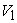is applied to stator terminals, a flux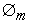is step up. This flux induces e. m. f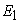in the stator winding. The flow of current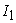causes voltage drops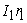and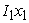in the stator winding.,,andare related to give motor equation. Figs. 3(b and c) are rotor circuit models. The mutual fluxinduces an e. m. f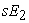in the rotor and a current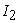flows.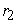and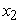are rotor resistance and leakage reactance at standstill (i. e. s =1). When the motor is operating at slip s, the leakage inductance is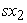as shown in Figure 3b. Figure 3c shows alternative rotor circuit model with mechanical load andin this model represents the line frequency current.Download asVeiw figureFigures index
Figure 4. Approximate equivalent circuit of 3phase induction motor

The 3-phase power input pi to an induction motor is given by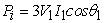(1)

Where,and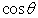are the per phase values of stator voltage and current and stator power factor respectively.

Stator copper losses is given by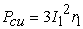(2)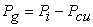(3)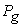is the power transferred, across the air gap, to the rotor. After rotor copper losses are subtracted from, the mechanical power developed,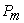is obtained. A part fromof power output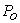is consumed as friction and windage and stray losses and the remainder is the power outputas can be seen in Figure 1.

Now,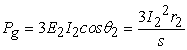(4)

Also,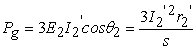(5)

Rotor Copper-loss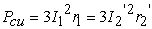(6)

From equations (4) and (6), Rotor Copper-loss = s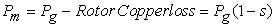(7)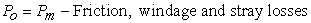(8)

The frequency of rotor current is very low. Therefore, power loss is very small and hence negligible.

The torque developed T is defined as the torque generated due to mechanical power developed by electro-mechanical power conversion process in the motor. The actual load torque available at the shaft is the torque developed less the windage and friction and stray losses.

Thus,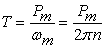(9)

Or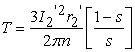(10)

Hint,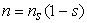The torque developed is proportional to the air gap power. The air gap poweris usually known as torque measured in synchronous watts. This indicates that if this power is divided by synchronous angular velocity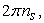Torque developed is obtained.

It can be ascertained from the approximate equivalent circuit in Figure 4 that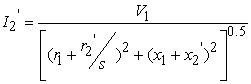(11)

Putting the value of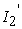from Eq. (11) into Eq. (10) yields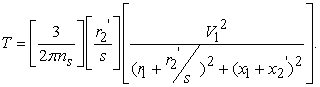(12)

### 5. Simulation Results

(a) MACHINE DATA

Table 1 below gives the machine data used for the simulation of the machine model for the analysis of speed control schemes.

#### Table 1. Machine details used in MATLAB codes execution for the various methods of speed controlDownload asPowerPoint Slide

Veiw figure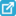View current table in a new window

(b) STEADY STATE BEHAVIOUR OF INDUCTION MACHINE

The steady state behaviour of the induction motors are graphically represented in Figure 5, Figure 6, Figure 7, Figure 8 and Figure 9 depending on the following variables: rotor resistance, line frequency, number of poles, stator voltage and stator voltage/line frequency.Download asVeiw figureFigures index
Figure 5. Torque-speed curves of 3 phase induction motor for different values of rotor resistanceDownload asVeiw figureFigures index
Figure 6. Torque-speed curves of 3-phase induction motor by varying line frequencyDownload asVeiw figureFigures index
Figure 7. Torque-speed curves of 3 phase induction motor by varying the number of poles.Download asVeiw figureFigures index
Figure 8. Torque-speed curves of 3-phase induction motor by varying the supply voltageDownload asVeiw figureFigures index
Figure 9. Torque-speed curves of 3-phase induction motor by varying stator voltage/line frequency

Figure 5 shows the torque-speed curve of an induction motor for different values of rotor resistances (R1,R2,R3,R4,R5). (From the graph it is observed that as the rotor resistance is increased, the speed decreases (or slip increases) while the electromagnetic torque remains constant. Figure 6 shows the torque-speed curve of an induction motor for different values of line frequencies. The plot depicts that as the frequency is increased, the speed increases (or slip deceases), consequently the electromagnetic torque decreases. Figure 7 shows the torque-speed curve of an induction motor for different values of poles. The plot depicts that as the pole is increased, the speed reduces (or slip increases), and thus the electromagnetic torque increases. Figure 8 shows the torque-speed curve of an induction motor for different values of stator voltages. The plot depicts that as the stator voltage is increased, the speed increases (or slip deceases), and thus the electromagnetic torque increases also. Finally, if the line voltage is changed in direct proportion to line frequency, the resulting torque-speed curves will be as shown in Figure 9.

### 6. Conclusion

Induction motors are not good machines for applications requiring considerable speed control. The normal operating range of a typical induction motor is confined to less than 5% slip, so the need for different speed controls arises. Torque-Speed characteristics for different methods of speed control of a three-phase Induction Motor were obtained and analyzed by developing MATLAB codes. In variable rotor resistance, the maximum electromagnetic torque is independent of rotor resistance, so, when the rotor resistance is increased, the maximum electromagnetic torque is unaffected but the speed at which it occurs can be directly controlled. In variable stator frequency, Increase in frequency increases the torque-speed relation and a decrease in frequency decreases the torque-speed relation of the motor. In variable stator voltage, varying the terminal voltage will vary the operating speed but with also a variation of operating torque. In terms of the range of speed variations, it is not significant hence this method is only suitable for small motors only. In constant volts/hertz control, the supply voltage as well as the supply frequency can be varied such that the ratio remains constant and the flux remains constant too. So, different operating zones for various speeds and torques can be got and also we can get different synchronous speed with almost same maximum torque. Thus the motor is completely utilized and also we have a good range of speed control. In pole changing method of speed control, to achieve higher speed and torque, lesser number of poles is required and to achieve lower speed and torque, the number of poles ought to be increased. Finally, it is recommended that any of the speed control method be implemented in a prototype.

### References

  Rashidi, F., Rashidi, M.,”Design of robust sliding mode speed control with fuzzy approach for induction motor”, IEEE international conference on industrial technology (ICIT03), pp. 27-30.In article  Stephen J. Chapman; Electric Machinery Fundamental; Second Edition; McGraw-Hill companies; 2012.In article  O. I. Okoro; Introduction to Matlab/Simulink for Engineers and Scientists; Second Edition; John Jacob’s Classic Publishers Ltd; 2008.In article  P. S. Bimbhra; Power Electronics; 3rd Edition; Khanna Publishers; 2005.In article  A. E. Fitzgerald et al; Electric machinery; Sixth Edition; McGraw publishers; 2003.In article  Shilpa V. Kailaswar, R. A. Keswani, “Speed Control of Three Phase Induction Motor by V/f Method for Batching Motion System” International Journal of Engineering Research and Application (IJERA) Vol. 3, Issue 2, March-April 2013, pp. 1732-1736.In article  Gourambika, M. S. Aspalli, “Speed Control of Three Phase Induction Motor by VVVF method using G7/A-1000 Drive” International Journal of Recent Technology and Engineering (IJRTE) Vol.-3, Issue-4, Sept 2014, pp. 43-49.In article  Chee- Mun Ong; Dynamic Simulation of Electric Machinery using Matlab and Simulink; Prentice hall PTR; 1998.In article  M.S.Aspalli, R. Asha, P.V. Hunagund, “Three Phase Induction Motor Drive Using IGBTs and Constant v/f Method” International Journal of Advanced in Electrical, Electronics and instrumentation Engineering vol. 1, Issue 5, November 2012, pp. 463-469.In article

### Appendix

MATLAB Code for Speed Control of three-phase Induction motor by varying the stator voltage. See Figure 7.

%================================================================

% M-file for Speed Control of three-phase Induction motor

% by varying the stator voltage.

%================================================================

s= 0:0.001:1; % slip

R1= 0.6; % stator resistance

X1= 1.1; % stator leakage reactance

R2p= 0.3; % rotor resistance referred to stator

X2p= 0.5; % rotor leakage reactance referred to stator

f= 50;P=4; % Supply frequency

Ws= 120*f/P; % synchronous speed

%Wm= -100:20:900; % rotor speed

Wm= (1-s).*Ws; % rotor speed

for Vs=400

A= (R1+(R2p./s).^2);

B= (X1+X2p).^2;

V= Vs./sqrt(3); % supply voltage

I1=(V.^2./(A+B)); % stator current

A1= 3./Wm;

A2= (1-s)./s;

A3= R2p.*I1;

Tem1= A1.*A2.*A3; % Electromagnetic torque

end

for Vs=440

A= (R1+(R2p./s).^2);

B= (X1+X2p).^2;

V= Vs./sqrt(3); % supply voltage

I1=(V.^2./(A+B)); % stator current

A1= 3./Wm;

A2= (1-s)./s;

A3= R2p.*I1;

Tem2= A1.*A2.*A3; % Electromagnetic torque

end

for Vs=480

A= (R1+(R2p./s).^2);

B= (X1+X2p).^2;

V= Vs./sqrt(3); % supply voltage

I1=(V.^2./(A+B)); % stator current

A1= 3./Wm;

A2= (1-s)./s;

A3= R2p.*I1;

Tem3= A1.*A2.*A3; % Electromagnetic torque

end

for Vs=500

A= (R1+(R2p./s).^2);

B= (X1+X2p).^2;

V= Vs./sqrt(3); % supply voltage

I1=(V.^2./(A+B)); % stator current

A1= 3./Wm;

A2= (1-s)./s;

A3= R2p.*I1;

Tem4= A1.*A2.*A3; % Electromagnetic torque

end

for Vs=520

A= (R1+(R2p./s).^2);

B= (X1+X2p).^2;

V= Vs./sqrt(3); % supply voltage

I1=(V.^2./(A+B)); % stator current

A1= 3./Wm;

A2= (1-s)./s;

A3= R2p.*I1;

Tem5= A1.*A2.*A3; % Electromagnetic torque

end

figure(1)

plot(Wm,Tem1,'r',Wm,Tem2,'b',Wm,Tem3,'g',Wm,Tem4,'k',Wm,Tem5,'m','linewidth',2.5)

grid on

xlabel('Speed [r.p.m]')

ylabel('Electromagnetic Torque [N-m]')

title('Graph of Electromagnetic Torque against Speed')

text(1250,31,'V1')

text(1250,37,'V2')

text(1250,44,'V3')

text(1250,48,'V4')

text(1250,52,'V5')

text(1100,15,'V5 > V4 > V3 > V2 > V1')